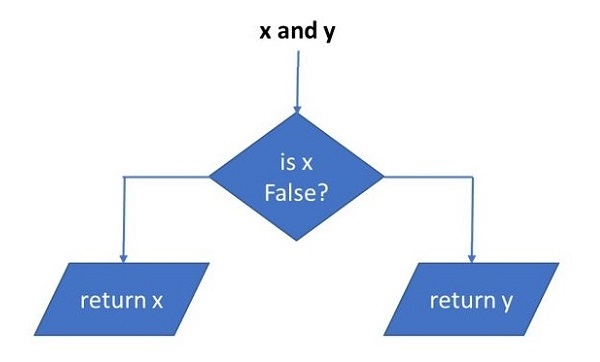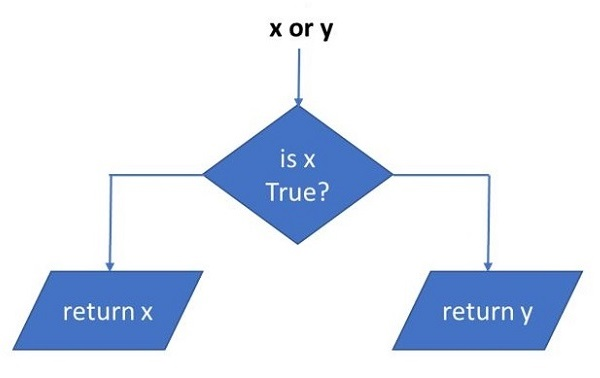# Python - Logical Operators

With Logical operators in Python, we can form compound Boolean expressions. Each operand for these logical operators is itself a Boolean expression. For example,

```age>16 and marks>80
percentage<50 or attendance<75
```

Along with the keyword False, Python interprets None, numeric zero of all types, and empty sequences (strings, tuples, lists), empty dictionaries, and empty sets as False. All other values are treated as True.

There are three logical operators in Python. They are "and", "or" and "not". They must be in lowercase.

### The "and" Operator

For the compound Boolean expression to be True, both the operands must be True. If any or both operands evaluate to False, the expression returns False. The following table shows the scenarios.

a b a and b
F F F
F T F
T F F
T T T

### The "or" Operator

In contrast, the or operator returns True if any of the operands is True. For the compound Boolean expression to be False, both the operands have to be False, ss the following table shows −

a b a or b
F F F
F T T
T F F
T T T

### The "not" Operator

This is a unary operator. The state of Boolean operand that follows, is reversed. As a result, not True becomes False and not False becomes True.

a not (a)
F T
T F

### How the Python interpreter evaluates the logical operators?

The expression "x and y" first evaluates "x". If "x" is false, its value is returned; otherwise, "y" is evaluated and the resulting value is returned.The expression "x or y" first evaluates "x"; if "x" is true, its value is returned; otherwise, "y" is evaluated and the resulting value is returned.Some use cases of logical operators are given below −

```x = 10
y = 20
print("x > 0 and x < 10:",x > 0 and x < 10)
print("x > 0 and y > 10:",x > 0 and y > 10)
print("x > 10 or y > 10:",x > 10 or y > 10)
print("x%2 == 0 and y%2 == 0:",x%2 == 0 and y%2 == 0)
print ("not (x+y>15):", not (x+y)>15)
```

It will produce the following output

```x > 0 and x < 10: False
x > 0 and y > 10: True
x > 10 or y > 10: True
x%2 == 0 and y%2 == 0: True
not (x+y>15): False
```

We can use non-boolean operands with logical operators. Here, we need to not that any non-zero numbers, and non-empty sequences evaluate to True. Hence, the same truth tables of logical operators apply.

In the following example, numeric operands are used for logical operators. The variables "x", "y" evaluate to True, "z" is False

```x = 10
y = 20
z = 0
print("x and y:",x and y)
print("x or y:",x or y)
print("z or x:",z or x)
print("y or z:", y or z)
```

It will produce the following output

```x and y: 20
x or y: 10
z or x: 10
y or z: 20
```

The string variable is treated as True and an empty tuple as False in the following example −

```a="Hello"
b=tuple()
print("a and b:",a and b)
print("b or a:",b or a)
```

It will produce the following output

```a and b: ()
b or a: Hello
```

Finally, two list objects below are non-empty. Hence x and y returns the latter, and x or y returns the former.

```x=[1,2,3]
y=[10,20,30]
print("x and y:",x and y)
print("x or y:",x or y)
```

It will produce the following output

```x and y: [10, 20, 30]
x or y: [1, 2, 3]
```